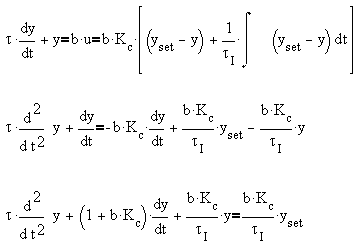Summary of Week 4

1. PID Controllers
Quick rules for selection:
1. Offset is acceptable ; use P-control
2. Fast process, offset undesirable: use PI-control
3. Slow process, offset undesirable: use PID-control

1. Time domain analysis of PID controlled process:
1. Substitute control algorithm for manipulated variable.
2. If integral mode is present, take derivatives of process equation to remove integration operation.
3. Rearrange equations so that only external variables remain on the right hand side of equation.
4. Procede with usual analysis, e.g. stability and steady state.

Example: Steady state analysis of  first order process with a PI controller, with constant set point.At steady state, y=yset, i.e. no offset.
1. Controller Tuning Methods
1. Ziegler-Nichols Method
1. Set controller to Proportional only control mode
2. Obtain the ultimate gain, Ku, and the corresponding ultimate period, Pu, by slowly increasing process gain until process sustains periodic oscillation
3. Using Ku and Pu, evaluate the controller parameters using the Ziegler-Nichols tuning table.
1. Autotuning Relay Method
1. Connect the feedback to an on-off controller:

2. If process gain is positive: U = uo+h (if y<yset), uo-h (otherwise)

If process gain is negative: U = uo-h (if yyset) uo+h (otherwise)

3. Wait until the process attains a limit cycle
4. Using the amplitude of the limit cycle, a, evaluate the approximate ultimate gain, Ku = (4/p)(h/a). Also record the period of oscillation of the limit cycle as the ultimate period, Pu.
5. Using the calculated Ku and Pu, use the Ziegler-Nichols tuning table.
1. Cohen-Coon Method (to be discussed next week)